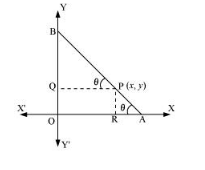# A rod of length 12 cm moves with its ends always touching the coordinate axes.

Question:

A rod of length 12 cm moves with its ends always touching the coordinate axes. Determine the equation of the locus of a point P on the rod, which is 3 cm from the end in contact with the x-axis.

Solution:

Let AB be the rod making an angle θ with OX and P (x, y) be the point on it such that AP = 3 cm.

Then, PB = AB – AP = (12 – 3) cm = 9 cm [AB = 12 cm]

From $\mathrm{P}$, draw $\mathrm{PQ} \perp \mathrm{OY}$ and $\mathrm{PR} \perp \mathrm{OX}$.In $\triangle \mathrm{PBQ}, \cos \theta=\frac{\mathrm{PQ}}{\mathrm{PB}}=\frac{x}{9}$

In $\triangle \mathrm{PRA}, \sin \theta=\frac{\mathrm{PR}}{\mathrm{PA}}=\frac{y}{3}$

Since, $\sin ^{2} \theta+\cos ^{2} \theta=1$,

$\left(\frac{y}{3}\right)^{2}+\left(\frac{x}{9}\right)^{2}=1$

Or, $\frac{x^{2}}{81}+\frac{y^{2}}{9}=1$

Thus, the equation of the locus of point $\mathrm{P}$ on the rod is $\frac{x^{2}}{81}+\frac{y^{2}}{9}=1$.